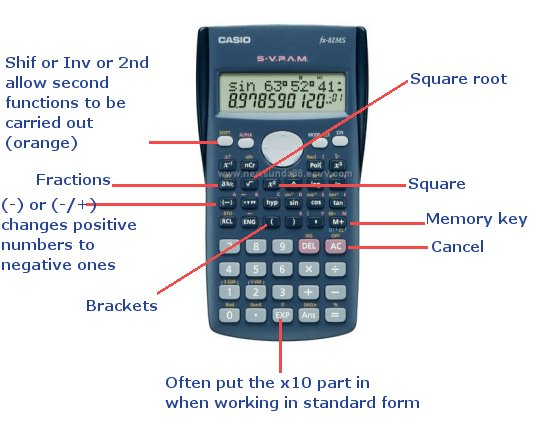HELP MATHS USING A CALCULATOR Return CALCULATOR KEYS This calculator is an imaginary to show you some of the most important keys:When you use a calculator remember BIDMAS We work out operations in this order: BRACKETS INDICES (Powers, roots, etc) DIVISION and MULTIPLICATION (working from left to right) ADDITION and SUBTRATION (working from left to right) BIDMAS! EXAMPLE: (2+3)x5 = 25 but 2+3x5 = 17, not 25, because without the brackets the multiplication is done first